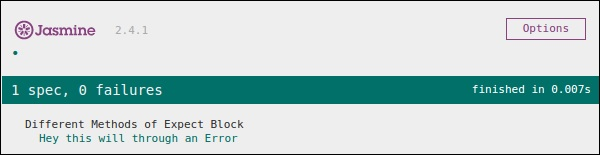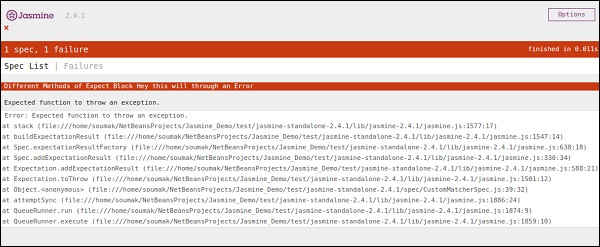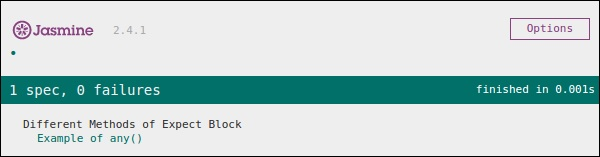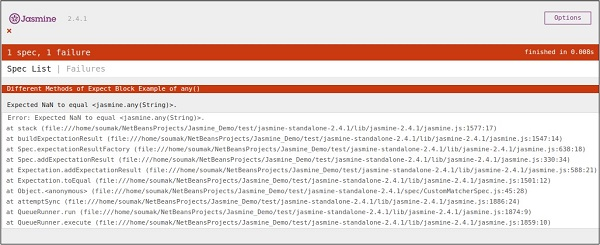# JasmineJS - Exception Check

Apart from different computational matchers, Jasmine provides some useful matchers to check exception of the program. Let us modify our JavaScript with the following set of code.

```var throwMeAnError = function() {
throw new Error();
};

describe("Different Methods of Expect Block", function() {
var exp = 25;

it ("Hey this will throw an Error ", function() {
expect(throwMeAnError).toThrow();
});
});
```

In the above example, we have created one method which deliberately throws an exception from that method and in the expect block we expect to catch the error. If everything goes well then this piece of code will yield the following output.Now, for this test case to fail, we need to omit that throw statement in the function throwMeAnError. Following is the code which will yield a red screenshot as an output since the code does not satisfy our requirement.

```var throwMeAnError = function() {
//throw new Error();
};

describe("Different Methods of Expect Block",function() {
var exp = 25;

it("Hey this will throw an Error ", function() {
expect(throwMeAnError).toThrow();
});
});
```

As can be seen, we have commented that line from where our method was throwing the exception. Following is the output of the above code on successful execution of the SpecRunner.html.## Jasmine.Any()

Any is the special matcher that is used when we are not sure about the output. In the following example, we will learn how this works. Let us modify the customerMatcher.js with the following piece of code.

```var addAny = function() {
var sum = this.currentVal;

for (var i = 0; i < arguments.length; i++) {
sum += arguments[i];
}

this.currentVal = sum;
return this.currentVal;
}

describe("Different Methods of Expect Block",function () {

it("Example of any()", function() {
});
});
```

Here we have declared one function that will give us the summation of the numbers provided as arguments. In the expect block, we are expecting that the result can be anything but it should be a Number.

As both 9 and 9 after sum yield 18 is a number, this test will pass and it will generate the following green screenshot as an output.Now let us change the code according to the following piece of code, where we are expecting a string type variable as an output of the function AddAny().

```var addAny = function() {
var sum = this.currentVal;

for(var i = 0; i < arguments.length; i++) {
sum += arguments[i];
}

this.currentVal = sum;
return this.currentVal;
}

describe("Different Methodsof Expect Block",function () {
it("Example of any()", function () {